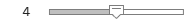# Interactive Calculus in Live Editor

This example shows how you can add interactive controls to solve a calculus problem in a live script.

An interactive control can be used to change the values of variables in your live script. To add a numeric slider, go to the Insert tab, click the Control button, and select Numeric Slider. For more information, see Add Interactive Controls to a Live Script.### Initialize Variables and Function

Evaluate the integral

`${\int }_{0}^{{x}_{Max}}c{x}^{2}\phantom{\rule{0.2777777777777778em}{0ex}}dx$`

using Riemann sum approximation.

A Riemann sum is a numerical approximation of the analytical integration by a finite sum of rectangular areas. Use the interactive slider bars to set the upper bound of the integral, the number of rectangles, and the constant factor of the function.

```syms x; xMax =4; numRectangles =30; c =2.5; f(x) = c*x^2; yMax = double(f(xMax));```

### Visualize the Area Under the Curve Using Riemann Sums

Plot the integrand `f`.

```fplot(f); xlim([0 xMax]); ylim([0 yMax]); legend({}, 'Location', 'north', 'FontSize', 20); title('Riemann Sum', 'FontSize', 20);```

Calculate the rectangular areas that approximate the area under the curve of the integral. Plot the rectangles.

```width = xMax/numRectangles; sum = 0; for i = 0:numRectangles-1 xval = i*width; height = double(f(xval)); rectangle('Position', [xval 0 width height], 'EdgeColor', 'r'); sum = sum + width*height; end text(xMax/10, yMax/3, ['Area = ' num2str(sum)], 'FontSize', 20);```### Calculate the Integral Analytically

Calculate the integral analytically. Use `vpa` to numerically approximate the exact symbolic result to 32 significant digits.

`fInt = int(f,0,xMax)`
```fInt =  $\frac{160}{3}$```
`vpa(fInt)`
`ans = $53.333333333333333333333333333333$`